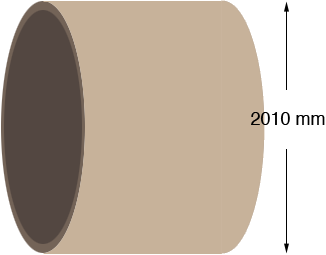SEARCH HOMEMath Central Quandaries & QueriesHow to calculate the plate size for rolling (2010mm OD)plate thick is 6mmIf I understand correctly you want to roll a metal plate into a cylinder of outside diameter 2010 mm.The perimeter or circumference of a circle is π × d where d id the diameter and hence the circumference of your cylinder is

π × d = π × 2010 = 6314.6 mm

Thus if you take a sheet of metal 6314.6 mm long and roll it into a cylinder the cylinder will have outside diameter of 2010 mm.

HarleyMath Central is supported by the University of Regina and The Pacific Institute for the Mathematical Sciences.